#### You may also like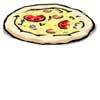### Pizza Portions

My friends and I love pizza. Can you help us share these pizzas equally?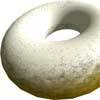### Doughnut

How can you cut a doughnut into 8 equal pieces with only three cuts of a knife?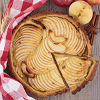### Pies

Grandma found her pie balanced on the scale with two weights and a quarter of a pie. So how heavy was each pie?

# More Fraction Bars

## More Fraction Bars

Look at these different coloured bars.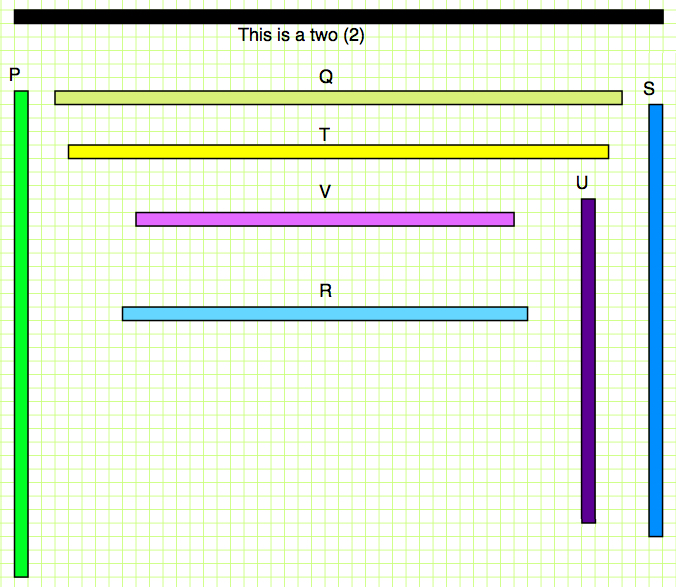Put the bars in size order - can you do it without cutting them out?

Now focus on this bar: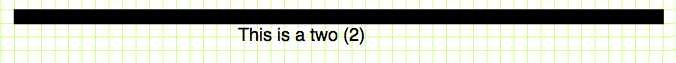This bar represents two wholes, or the number 2. You might find it easier to think of it as two bars which are each 'one whole' that have been stuck together.

We are thinking about all the other coloured bars as fractions of this bar, so we are thinking about them as fractions of 2.

For example, look at Bar S below: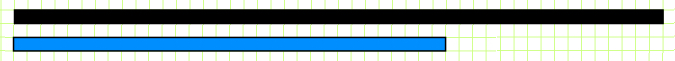Drawing lines helps us measure it against the black bar: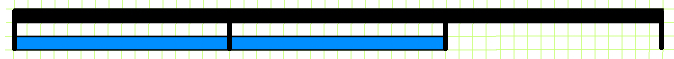What fraction of the black bar is Bar S?

Go through each of the other coloured bars and compare them to the black bar. What fraction of 2 is each bar?

Write down your ideas for each bar. For example, you could write:
Bar S is two thirds of the black bar.
or
Bar S represents $\frac{2}{3}$ of 2.
or
Bar S is 1$\frac{1}{3}$.

Can you work out how we came up with these three ideas?

When you've worked out what fraction of 2 each bar represents, have a go at the next challenge here.

### Why do this problem?

This activity gives pupils the opportunity to practise ordering and comparing fractions. It extends the idea of finding a fraction of a whole to finding a fraction of 2. Working together on this task will encourage pupils to build their fraction vocabulary.

### Possible approach

Begin by showing some rods of different lengths on the IWB. Put a row of smaller rods together and show that this is the same length as a larger rod. Can pupils work out what fraction of the larger rod each smaller rod is? What if the larger rod represents 2 - what fraction does the smaller rod represent now?

In pairs or small groups, children should have access to multilink cubes, making each bar of the same colour throughout. Otherwise, pupils can use this sheet (wordpdf) along with some squared paper to make paper copies of each bar. It may be helpful for children to make multiple versions of the same bar in order to see that these can be fitted together to be the same length as the black bar.

Give pupils some time to investigate the order of size of the bars, and what fraction each bar represents. Bring the whole class together for a plenary - can the groups explain their choices? There may be disagreements between different groups and this can lead to a healthy discussion, encouraging each group to give reasons for their suggestion.

### Key questions

Why is what you've chosen true?
Can you think of a way of writing that down?
"P is 1$\frac{1}{5}$ of R as P = 36 and R = 30."